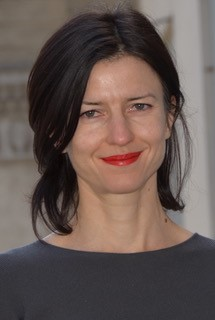distinguished lecture series presents

### UC Berkeley

Particle Physics

###### Visit

Tuesday, May 12, 2020 to Thursday, May 14, 2020

###### Location

All virtual lectures will be hosted on Zoom: https://ucla.zoom.us/j/9264073849

# Website

## Knot categorification from mirror symmetry

###### abstracts
In the series of lectures, I will describe two approaches to categorifying quantum link invariants which work uniformly for all simple Lie algebras, and originate from geometry and from string theory. Many ingredients that go into the story have been found by mathematicians earlier, but physics spells out how they should be put together for a uniform framework for the knot categorification problem. One of the important aspects of both approaches is that the fact that decategorification gives the quantum link invariants one set out to categorify, becomes manifest. The first approach is based on derived categories of coherent sheaves on resolutions of slices in affine Grassmannians. While some elements of it are familiar to mathematicians, others are new. The second approach is perhaps more surprising. It uses symplectic geometry and is related to the first by two dimensional (equivariant) mirror symmetry. Unlike previous symplectic geometry based approaches, it produces a bi-graded homology theory. In both cases, mirror symmetry, and techniques developed by physicists and mathematicians to understand it play a crucial role. I will also explain the relation to another string theory based approach, due to Witten.
In the series of lectures, I will describe two approaches to categorifying quantum link invariants which work uniformly for all simple Lie algebras, and originate from geometry and from string theory. Many ingredients that go into the story have been found by mathematicians earlier, but physics spells out how they should be put together for a uniform framework for the knot categorification problem. One of the important aspects of both approaches is that the fact that decategorification gives the quantum link invariants one set out to categorify, becomes manifest. The first approach is based on derived categories of coherent sheaves on resolutions of slices in affine Grassmannians. While some elements of it are familiar to mathematicians, others are new. The second approach is perhaps more surprising. It uses symplectic geometry and is related to the first by two dimensional (equivariant) mirror symmetry. Unlike previous symplectic geometry based approaches, it produces a bi-graded homology theory. In both cases, mirror symmetry, and techniques developed by physicists and mathematicians to understand it play a crucial role. I will also explain the relation to another string theory based approach, due to Witten.
In the series of lectures, I will describe two approaches to categorifying quantum link invariants which work uniformly for all simple Lie algebras, and originate from geometry and from string theory. Many ingredients that go into the story have been found by mathematicians earlier, but physics spells out how they should be put together for a uniform framework for the knot categorification problem. One of the important aspects of both approaches is that the fact that decategorification gives the quantum link invariants one set out to categorify, becomes manifest. The first approach is based on derived categories of coherent sheaves on resolutions of slices in affine Grassmannians. While some elements of it are familiar to mathematicians, others are new. The second approach is perhaps more surprising. It uses symplectic geometry and is related to the first by two dimensional (equivariant) mirror symmetry. Unlike previous symplectic geometry based approaches, it produces a bi-graded homology theory. In both cases, mirror symmetry, and techniques developed by physicists and mathematicians to understand it play a crucial role. I will also explain the relation to another string theory based approach, due to Witten.
###### Lecture 3
2300 Murphy Hall - Box 951438 - Los Angeles, CA 90095-1438 © 2018